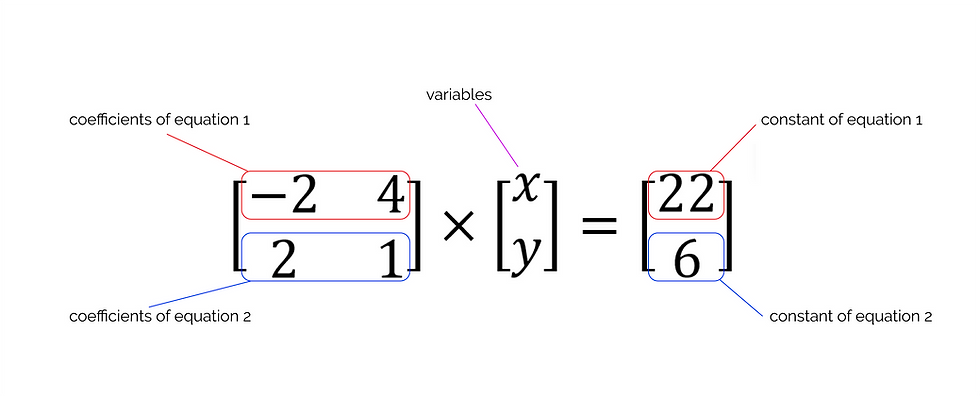cum
top of page
Search

# CSEC Mathematics: Solving Linear Simultaneous Equations Using Matrices

You should already be familiar with simultaneous equations; several equations containing two or more variables which have the same values in each equation. For example:

3x + 4y = 24

4x + 3y = 22

There are several methods for solving simultaneous equations, including substitution, the graphical method and elimination. In this lesson, we will discuss how to solve simultaneous linear equations (like the ones above) using matrices.

(Before we start, you should be familiar with matrix multiplication and finding the inverse of a matrix)

Solving Simultaneous Equations Using Matrices

Find the values of x and y using matrices.

4y - 2x = 22 (equation 1)

2x + y = 6 (equation 2)

First, you write the equations in the form ax + by = c:

-2x + 4y = 22

2x + y = 6

Then, you write the coefficients, variables and constants using matrices:Why do we write it like this? Well, this is all in line with dot multiplication, which is how we multiply matrices. So, if we were to multiply the matrix equation above:We get back the original equation.

Getting back to the procedure, you now want to find the values of x and y. The matrix containing x and y is multiplied by the matrix containing the coefficients, so, it would make sense to divide both sides by the coefficient matrix- but alas, you cannot divide matrices. Instead, you multiply each side by the inverse of the coefficient matrix to find the values of x and y.

As you know, the inverse of a matrix is found by multiplying the reciprocal of the determinant by the adjoint of the matrix.

Determinant = (a × d) - (b × c)

You find the adjoint of a 2×2 matrix by switching the places of the a and d terms and multiplying the b and c terms by -1.Now that we know the inverse of the coefficient matrix, we multiply both sides by it.(We can cancel out the coefficient matrix and its inverse since they will multiply to form an identity matrix, which, as you know, will multiply another matrix to give the original matrix, changing nothing)So, x = 1/5 and y = 28/5.

We can check this by substituting the x and y values into both equations to make sure:

4y - 2x = 22

4(28/5) - 2(1/5) = 22

112/5 - 2/5 = 22

110/5 = 22

22 = 22

2x + y = 6

2(1/5) + 28/5 = 6

2/5 + 28/5 = 6

30/5 = 6

6 = 6